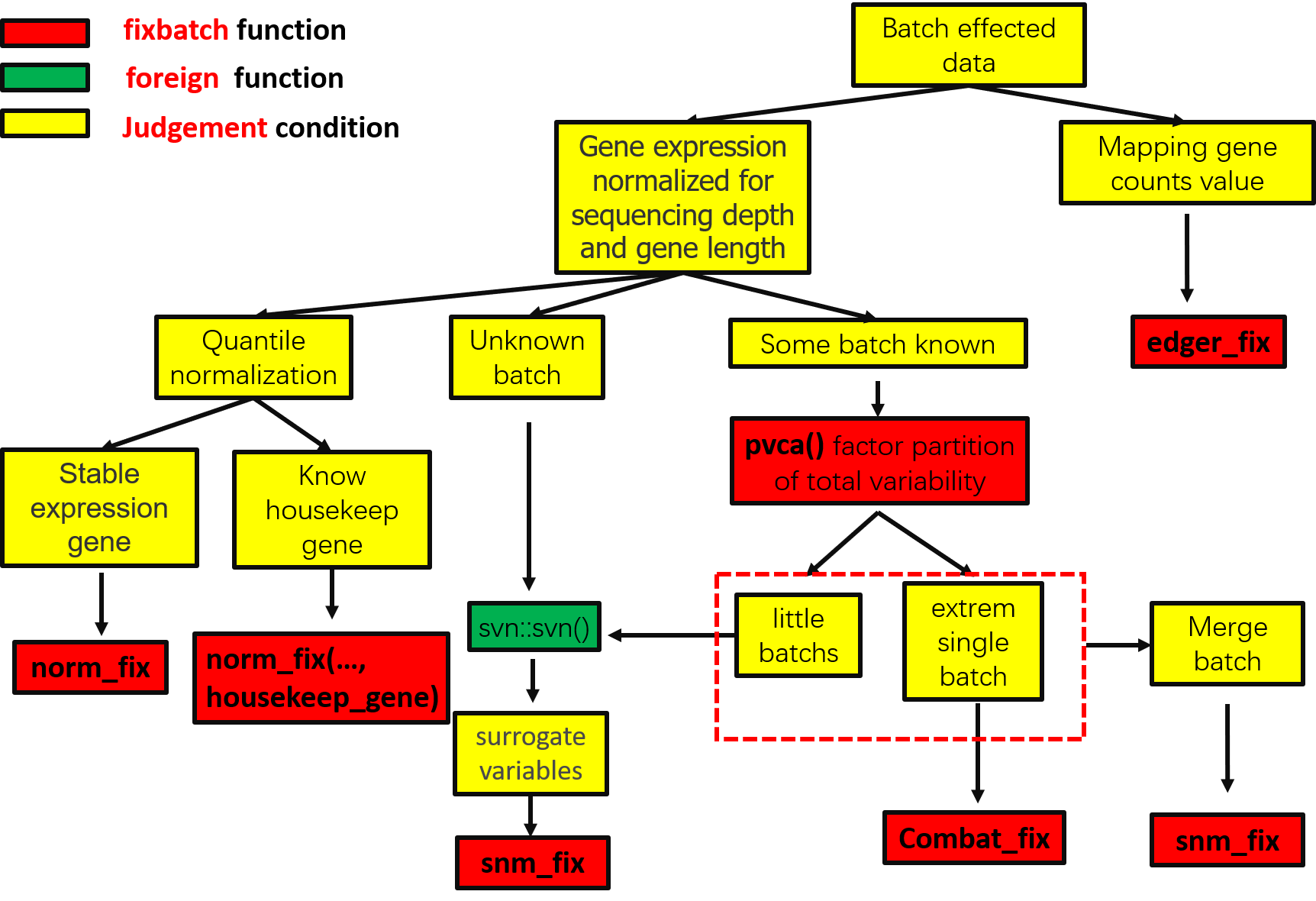fixbatch矫正思路

#记得更新下xbox
#devtools::update_packages("xbox")
library(xbox)
library(ggplot2)
library(hexSticker)
#构建绘图数据
dat <- tibble::tribble(
~id, ~value,  ~x, ~y,
"right", "grey",   1, 1L,
"right", "grey",   1, 8L,
"right", "grey",   3, 7L,
"right", "grey",   3, 2L,
"left", "grey", 3.5, 7L,
"left", "grey", 3.5, 2L,
"left", "grey", 5.5, 1L,
"left", "grey", 5.5, 8L
)

#中国风配色
back_color = xbox::need_colors("three2")
bord_color = xbox::need_colors("two4")
#绘图
ggplot(dat, aes(x = x, y = y)) +
geom_polygon(aes(fill = id, group = id)) +
geom_point(aes(x = 3.25-0.5,y = 4.5),color =back_color,size = 6) +
geom_point(aes(x = 3.25+0.5,y = 4.5),color =back_color,size = 6) +
scale_fill_manual(values = xbox::need_colors("two5")) ->p
p <- p + theme_void() + theme_transparent() + theme(legend.position="none")
#生成hex sticker
sticker(p, package="fixbatch", p_color = bord_color ,p_size=20, p_y = 0.4,s_x=1, s_y=1, s_width=1.5, s_height=1.5/1.3,
h_fill=back_color, h_color = bord_color, filename="ggplot21.png")logo